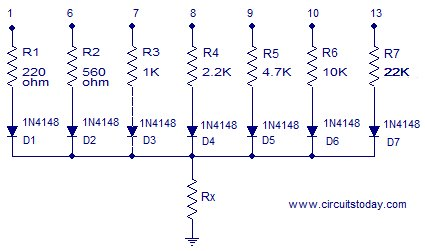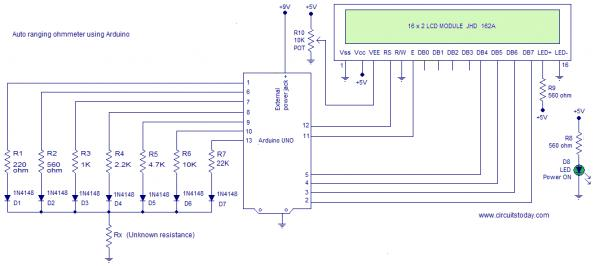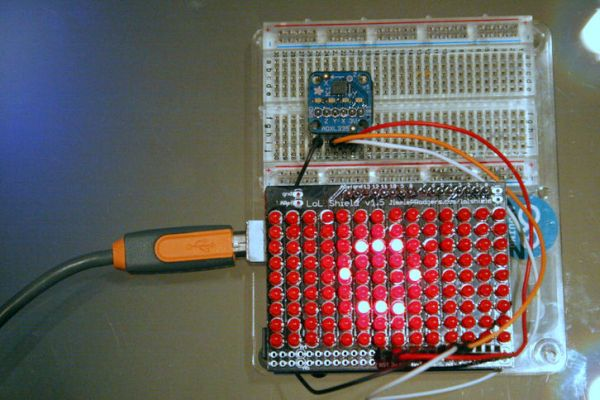# OhmMeter using Arduino – with Auto Ranging Feature

### Auto ranging ohmmeter using arduino.

This article is about a simple auto ranging ohmmeter using arduino. The measured resistance is displayed using a 16×2 LCD display. The circuit is sufficiently accurate and uses minimum number of external components possible. Before going into the details of this project, lets have a look at the basic resistance measurement method.#### Resistance measurement.

The figure above shows the circuit diagram of a simple resistance measurement scheme. Rx is the resistance to be measured. R1 is the input resistance. i is the current passing through the loop and 5V is the supply voltage. To find the unknown resistance Rx, the voltage across Rx is measured first. let the voltage across R1 be VR1. Then VR1=5-Vx.  The current i=VR1/R1=(5-Vx)/R1. Since R1 and Rx are connected in series, the current through them will be equal. So the unknown resistance Rx= Vx/i. The voltage across the unknown resistance is measured using the ADC of the arduino. To be precise, analog channel A5.

Anyway this method have a drawback. If there is great difference between the input resistance and the Rx, the result will be extremely inaccurate. This is because almost all of the input voltage will drop across the larger resistance and this provides very less information.

• Suppose R1=10K and Rx=100 ohm. Then the voltage across R1 will be 4.95v and voltage across Rx will be 50mV and this gives less information. The sensitivity of the arduino is 4.889mV. So when we read 50mV  using the arduino ADC the result will be 10. When converted it into voltage the result will be 10 x 4.889mV =48.89mV.  Then Rx= 0.0488/((5V-48.89mV)/10000) = 98.7 ohm.
• Suppose R1=10 and Rx=220 ohm. Then the voltage across R1 will be 4.89V and voltage across Rx will be 107mV. The corresponding digital reading will be 21. When we convert it into voltage the result will be 21 x 4.889mV=102mv. Following the calculations used in the previous case, Rx=208 ohm.
READ  EEVblog #669 – FLIR TG165 Thermal Imager Teardown

In the above two cases you can see accuracy issues. The most accurate result occurs when the Rx and R1 are as close as possible.#### Auto ranging.

A scheme for estimating the value of Rx roughly and then putting a matching resistor in place of R1 is what we  need here and this method is called auto ranging. The circuit given below demonstrates auto ranging.

For more detail: OhmMeter using Arduino – with Auto Ranging Feature

#### This Post / Project can also be found using search terms:

• OhmMeter using Arduino – with Auto Ranging Feature
• arduino ohmmeter
• arduino омметр
• auto ranging ohmmeter